Induced EMF

Homework Statement

[/B]
A conducting square frame of side a and a long straight wire carrying current I and located in the same plane as shown in the figure. The frame moves to the right with a constant velocity v. The emf induced in the frame will be proportional to

Homework Equations

[/B]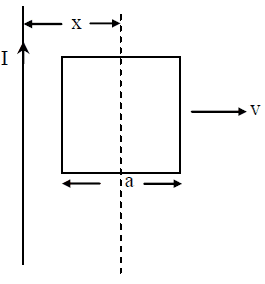The Attempt at a Solution

My teacher solved this in following manner

EMF induced in one side =B1vl
EMF induced in the other side=B2vl
EMF in the frame=B1vl - B2vl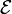∝ B1 - B2
Since B1∝1/r.I don't understand from where the below formula is coming?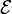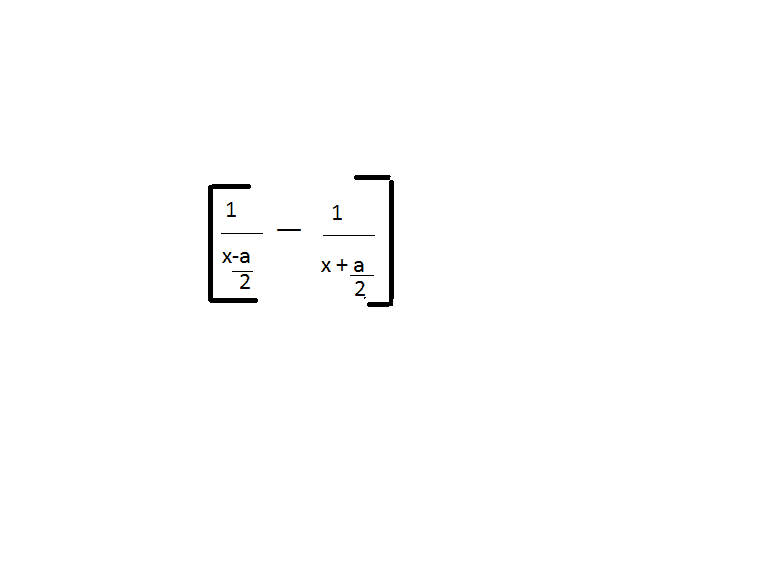[/B]

Last edited by a moderator:

Doc Al
Mentor
Since B1∝1/r.I don't understand from where the below formula is coming?
r is the distance between the current-carrying wire and each side. Express that distance in terms of the given x and a.

. Express that distance in terms of the given x and a.
It was about proportionality.But My teacher gave full formula for magnetic field is B2= μ0 I /2 pi(X+a/2)
B1=μ0 I/2 pi(X - a/2)
But μ0 I/2 pi r is the formula for magnetic field due to a wire which is infinitely long from both side which is not the case here so I know it doesn't matter while taking proportionality into account but I wan to clarify this .

For clarity, only the frame of the square is conducting - right? We don't have a square conductor - right? Just a current loop that is the shape of a square?

Doc Al
Mentor
But μ0 I/2 pi r is the formula for magnetic field due to a wire which is infinitely long from both side which is not the case here
What do you mean "from both sides"? The formula is for the magnetic field from an infinitely long current-carrying wire, which is a reasonable approximation here. Only one wire is involved. It has nothing to do with the length of the side of the square, if that's what you mean.

What do you mean "from both sides"?
In my book there are 2 separate cases given
one which involves a wire of infinite length from both sides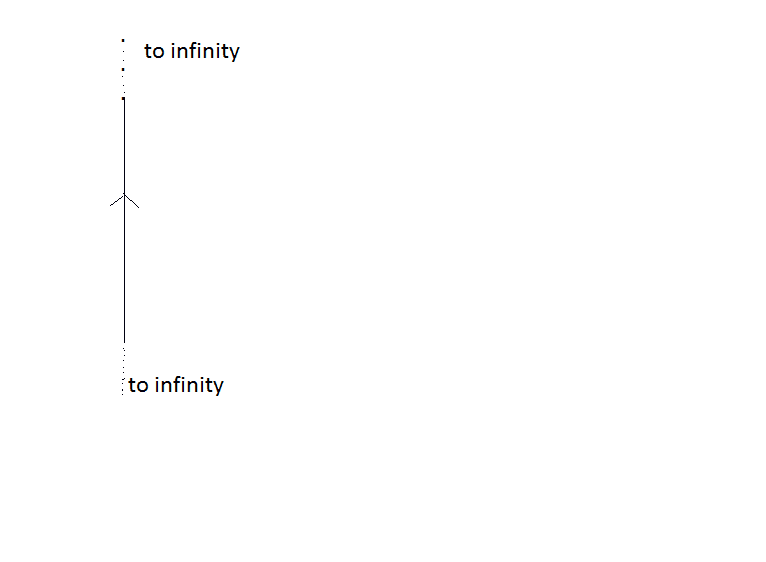And the second which has a wire with one starting point and the other end going to infinity.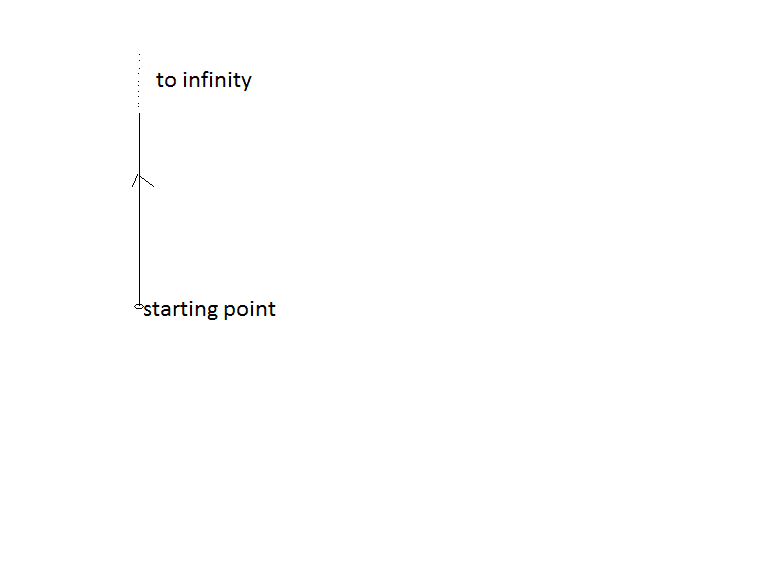Last edited:
which is a reasonable approximation here.
But it is not mentioned in the question that wire is infinitely long.
It has nothing to do with the length of the side of the square,
sir,I am aware of this fact.

Doc Al
Mentor
In my book there is 2 separate cases given
one which involves a wire of infinite length from both sides

And the second which has a wire with one starting point and the other end going to infinity.
Ah, I see what you mean.

Now, looking at the diagram you posted originally, which case do you think they mean?

Doc Al
Mentor
But it is not mentioned in the question that wire is infinitely long.
You are told that it is a long straight wire. So it is reasonable to assume you can treat it as infinitely long, for the purposes of this problem.

it is reasonable to assume you can treat it as infinitely long, for the purposes of this problem.
Don't we have any formula for magnetic field due to normal conventional wire we see in our daily life ?

Don't we have any formula for magnetic field due to normal conventional wire we see in our daily life ?

Finite-length wires are a pain in magnetics. Think about the integration involved (especially if you have to deal with asymmetries). Blah! The fields produced by infinitely-long current-carrying conductors behave very nicely mathematically.

Remember, you can look at this from the inertial frame of the magnetic field or from the inertial frame of the square loop.

I would also advise considering each segment of the square loop separately.

The resulting expression for the emf should make sense. Think about these quantities: ##\mu##, ##I##, ##a##, ##v##, and ##x##. Intuitively, how would increasing or decreasing these quantities affect your emf?

•gracy
rude man
Homework Helper
Gold Member
But it is not mentioned in the question that wire is infinitely long.
.
If a problem says "a long wire" you should assume they mean "infinitely long".

•gracy
rude man
Homework Helper
Gold Member
Don't we have any formula for magnetic field due to normal conventional wire we see in our daily life ?
Yes. It's called the Biot-Savart law.
For example, you could have a closed square loop carrying current. In theory you can use B-Savart to compute the mag field anywhere. In practice the math in this case becomes prohibitively difficult except for calculating B at the center of the square.

Yes. It's called the Biot-Savart law.
For example, you could have a closed square loop carrying current. In theory you can use B-Savart to compute the mag field anywhere. In practice the math in this case becomes prohibitively difficult except for calculating B at the center of the square.

I do not agree that you want to calculate B at the center of the square. There is no conductor there, and we are trying to find the emf in the circuit.

This problem is a great problem for studying the so-called motional electric field intensity.

Also, if you have never studied the special theory of relativity, once you master the concepts of this problem, then it would be a great time to read Einstein's paper - at least the first page of it.

Attachments

• On the Electrodynamics of Moving Bodies - Einstein's Special Theory of Relativity.pdf
226 KB · Views: 130
Doc Al
Mentor
Don't we have any formula for magnetic field due to normal conventional wire we see in our daily life ?
First learn to solve the simple problems, then you can add more complexity by considering finite lengths of wire. (As rude man said, that would involve the Biot-Savart law and calculus.)

Doc Al
Mentor
I do not agree that you want to calculate B at the center of the square. There is no conductor there, and we are trying to find the emf in the circuit.
He was not suggesting that for this problem. He was giving an example of the complications involved with applying Biot-Savart.

He was not suggesting that for this problem. He was giving an example of the complications involved with applying Biot-Savart.

I see.

andrevdh
Homework Helper
The magnetic field at a distance R form the wire with a current I in it is
B = k I/R
where k is some constant. The magnetic field at the point where the two conductors are
depends on where they are during the motion, x- a/2 for the one on the left and x+a/2 for
the one on the right.

Attachments

•gracy
andrevdh
Homework Helper
Since I is the same for both B1 and B2 the formula becomes even simpler

B = k 1/R